# Partition of Variation

library(POV)

# Variance components

## Introduction to variance components

### Single factor

Assume a response that looks like this:

hist(dt2$Response, main = "")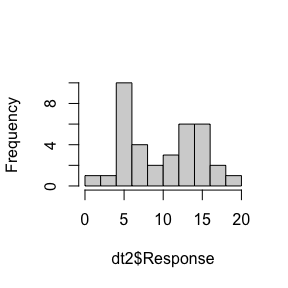Figure 1 Distribution of example response sd(dt2$Response)
#>  4.775548
var(dt2$Response) #>  22.80586 In variance components analysis the effect of one or more factors on the response is measured. Since the calculation of standard deviation involves a square root, all math is done using variance and converted to a standard deviation only for the final answer. The factor ‘group’ is introduced and analyzed using ANOVA: plot(factor(dt2$Group),dt2$Response, xlab="Group", ylab="Response")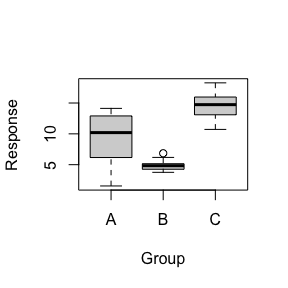Figure 2 Visualizing variance components As the level of the factor ‘group’ changes, both the response mean and standard deviations change (are variable). The variation in the means and the variation of the standard deviations are two components of the total variation. The first is the between variation and the second is the within variation. There is a minimum amount of variation present in all groups (the level of B) and there is more variation at other levels of ‘group’. This minimum amount of variation that is present in all groups is called the common variation since it is common to all groups. Mathematically the variance components in this example are: $SD_{Overall} = \sqrt{SD_{Between Group}^2+SD_{Within Group}^2+SD_{Common}^2 }$ ### Multiple factors In the multivariate case, the factor structure becomes important: #### Nested Nested factors are factors where some levels for one factor can only occur in combination with a specific level of another factor. Nested happens in manufacturing when product that comes from Machine A can only go to certain downstream machines and Machine B goes to other downstream machines. In this case the downstream machines are nested in the upstream machines. An example is analytical equipment (metrology) nested within laboratories.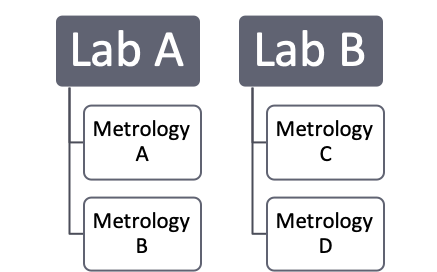Figure 3 Nested factor structure Metrology A and B only exist in Lab A and will never be combined with product from Lab B. In the case of nested data the variance components that can be calculated are: $SD_{Overall}=\sqrt{SD_{Between Lab}^2 + SD_{Between Metrology[Lab]}^2 + \\ SD_{Within Lab}^2 + SD_{Within Metrology[Lab]}^2 + SD_{Common}^2 }$ #### Crossed Crossed factors are structured experiments where all levels of factor 1 have been tested at all levels of factor 2. This structure allows us to see if the effect of factor 2 on the response depends on the level of factor 1, this is called a combination or interaction effect. These can only be calculated when factor combinations have been run correctly. Interaction effects are mathematically notated in the form: factor 1 * factor 2.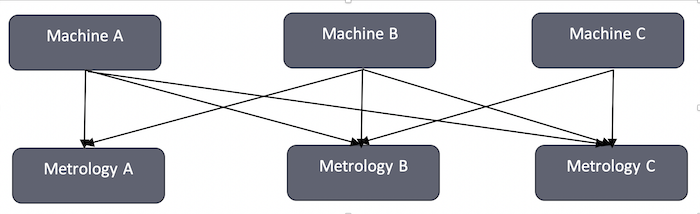Figure 4 Crossed factor structure In the case of a 2 factor crossed study the variance components are: $SD_{Overall}=\sqrt{SD_{Between Machine}^2+SD_{Between Metrology}^2+SD_{Between Machine*Metrology}^2+\\SD_{Within Machine}^2+SD_{Within Metrology}^2+SD_{Within Machine*Metrology}^2+\\SD_{Common}^2}$ ## Variance components estimation methods ### Partition of Variation (POV) POV was invented by Thomas A. Little in 1993 for the analysis of semiconductor data for hard drive manufacturing. In 2015 Thomas A. Little and Paul Deen collaborated on expanding the functionality of the POV engine with a full suite of Measurement System Analysis (MSA) tools. The POV engine is currently publicly available as a JSL script for use in JMP statistical software from SAS and can be found on the website POV is an exact method because it uses sums of squares to precisely quantify the sample variance components. The data used here contains one response and the factors Machine and Metrology. A quick view of the data is provided in three graphs: hist(dt$Response, main = "")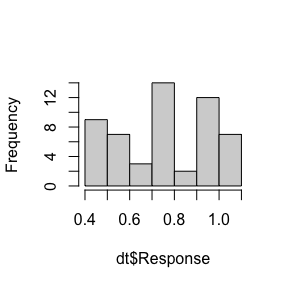plot(factor(dt$Machine),dt$Response, xlab="Machine", ylab="Response")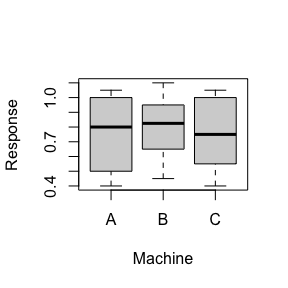plot(factor(dt$Metrology),dt$Response, xlab="Metrology", ylab="Response")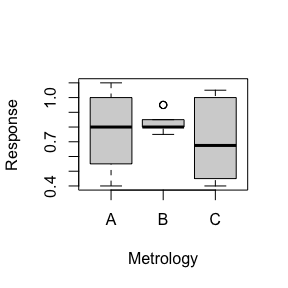Figure 5 Three part data overview

POV uses generalized linear regression, using the lm function, to calculate the sum of squares for the model and the error.

anova(lm(dt$Response ~ dt$Machine * dt$Metrology)) #> Analysis of Variance Table #> #> Response: dt$Response
#>                         Df  Sum Sq  Mean Sq F value Pr(>F)
#> dt$Machine 2 0.01861 0.009306 0.1825 0.8338 #> dt$Metrology             2 0.09694 0.048472  0.9508 0.3941
#> dt$Machine:dt$Metrology  4 0.05861 0.014653  0.2874 0.8846
#> Residuals               45 2.29417 0.050981

Table 1 Total ANOVA

$Var_{Between total}=\frac{SS_{Model terms}}{SS_{Total}} * Var_{Total} *\frac{N-1}{N} =\frac{0.1741667}{2.4683334}*0.04571=0.003225$ $Var_{Within total}=\frac{SS_{Error}}{SS_{Total}} * Var_{Total} *\frac{N-1}{N}= \frac{2.294167}{2.4683334}*0.04571=0.042485$

Then the individual sum of squares are used to calculate the between factor effects as a fraction of the total between variance. The between variance components are:

$Var_{Between Machine}=\frac{SS_{Machine}}{SS_{Total}} * Var_{Between Total}= \frac{0.01861111}{0.17416666}*0.003225=0.000345$

$Var_{Between Metrology}=\frac{SS_{Machine}}{SS_{Total}} * Var_{Between Total}= \frac{0.09694444}{0.174167}*0.003225=0.001795$

$Var_{Between Machine*Metrology}=\frac{SS_{Machine}}{SS_{Total}} * Var_{Between Total}= \frac{0.05861111}{0.174167}*0.003225=0.001085$

Then the response is summarized into the variance, grouped by the factors. Because r always reports the sample variance, this is upscaled to the population variance by multiplying by (N-1)/N.

VarTable
#>   Machine Metrology  rowVariance rowN       popVar
#> 1       A         A 0.0880000000    6 0.0733333333
#> 2       B         A 0.0506666667    6 0.0422222222
#> 3       C         A 0.0617500000    6 0.0514583333
#> 4       A         B 0.0000000000    6 0.0000000000
#> 5       B         B 0.0044166667    6 0.0036805556
#> 6       C         B 0.0006666667    6 0.0005555556
#> 7       A         C 0.0970000000    6 0.0808333333
#> 8       B         C 0.0356666667    6 0.0297222222
#> 9       C         C 0.1206666667    6 0.1005555556

Table 3 Variance table

The common variance is equal to 0 as defined by the combination of Machine A, and Metrology B. Using generalized regression to fit the population variance produces another set of sequential sum of squares for the within variance components.

anova(lm(VarTable$popVar ~ VarTable$Machine * VarTable$Metrology)) #> Warning in anova.lm(lm(VarTable$popVar ~ VarTable$Machine * #> VarTable$Metrology)): ANOVA F-tests on an essentially perfect fit are unreliable
#> Analysis of Variance Table
#>
#> Response: VarTable$popVar #> Df Sum Sq Mean Sq F value Pr(>F) #> VarTable$Machine                     2 0.0013435 0.0006718
#> VarTable$Metrology 2 0.0079154 0.0039577 #> VarTable$Machine:VarTable\$Metrology  4 0.0018478 0.0004620
#> Residuals                            0 0.0000000

Table 4 Within ANOVA

The common variance is subtracted from the total within and the remainder is used for the within components using the same calculation that produced the between variance components.

$Var_{Within Machine}=\frac{SS_{Machine}}{SS_{Total}} *Var_{Within Total}-Var_{Common}= \frac{0.00134353}{0.01110672}*0.042485=0.005139$ $Var_{Within Metrology}=\frac{SS_{Metrology}}{SS_{Total}} *Var_{Within Total}-Var_{Common}= \frac{0.00791538}{0.01110672}*0.042485=0.030277$ $Var_{Within Machine*Metrology}=\frac{SS_{Machine*metrology}}{SS_{Total}} *Var_{Within Total}-Var_{Common}=\\ \frac{0.00184781}{0.01110672}*0.042485=0.007068$

The complete set of variance components are:

POV(Response ~ Machine * Metrology, dt, Complete = TRUE)
#>                                 Variance     StdDev % of total
#> Between Total               0.0032253086 0.05679180   7.056043
#>   Between Machine           0.0003446502 0.01856476   0.753995
#>   Between Metrology         0.0017952675 0.04237060   3.927526
#>   Between Machine:Metrology 0.0010853909 0.03294527   2.374522
#> Within Total                0.0424845679 0.20611785  92.943957
#>   Within Machine            0.0051391764 0.07168805  11.243033
#>   Within Metrology          0.0302773059 0.17400375  66.237995
#>   Within Machine:Metrology  0.0070680857 0.08407191  15.462929
#>   Common                    0.0000000000 0.00000000   0.000000
#> Total                       0.0457098765 0.21379868 100.000000

Table 5 Variance components